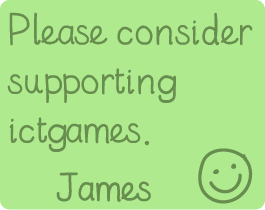# Finger Counter

### Find multiplication facts using counting.

Learning Objectives:
• Year 1: To solve one-step problems involving multiplication (and division), by calculating the answer using concrete objects, pictorial representations and arrays with the support of the teacher.
• Year 2: To recall and use multiplication and division facts for the 2, 5 and 10 multiplication tables, including recognising odd and even numbers.
A tool for modelling use of counting to find multiplication number facts. This is the step before knowing the facts by heart, which is much faster and more preferrable.

### Instructions:

1. Choose a times table from the top menu
2. Decide how many times you need
3. Keep pressing fingers until you reach the desired multiplication fact

Have fun!Made using theandlibraries.